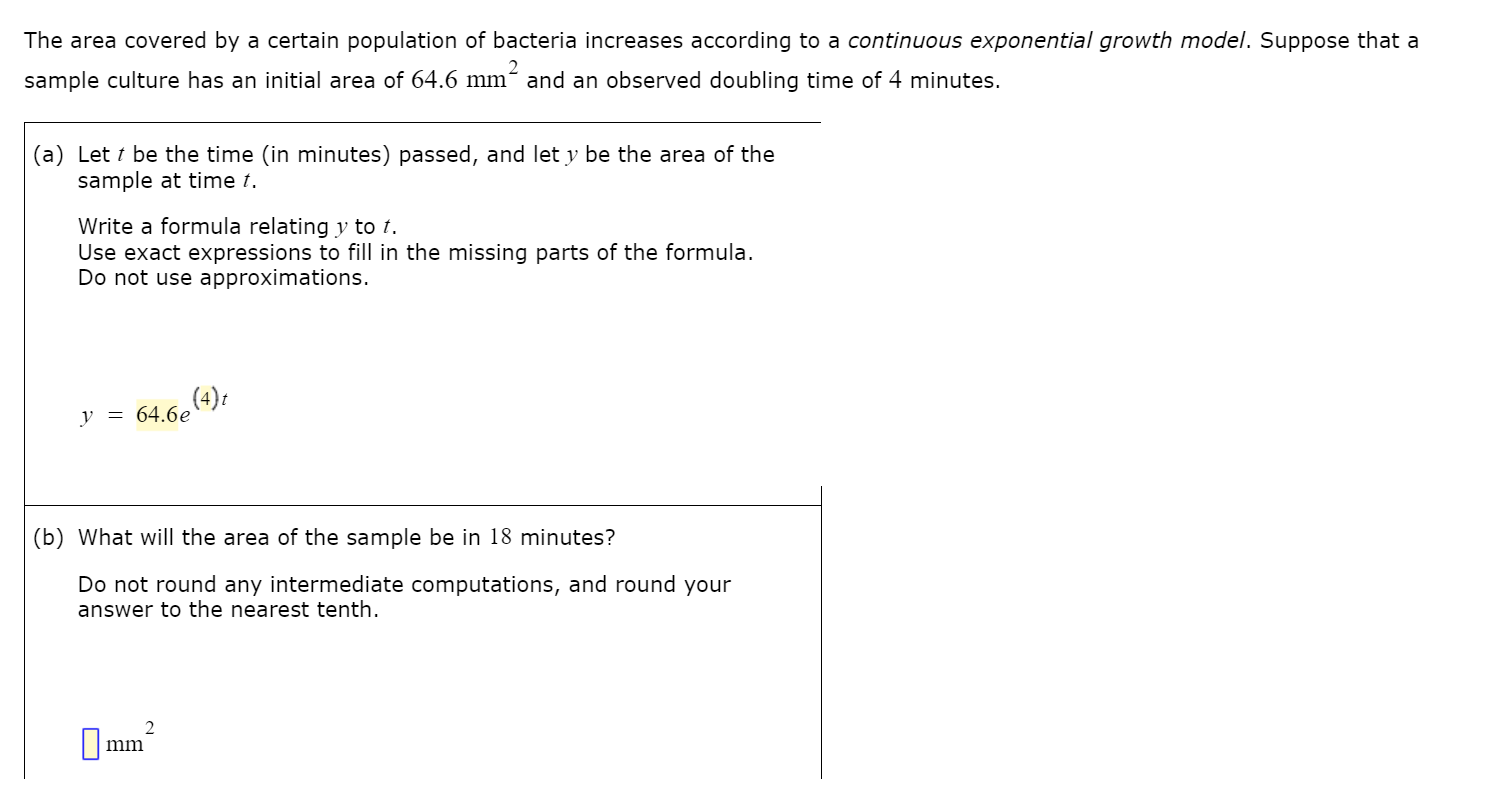# The area covered by a certain population of bacteria increases according to a continuous exponential growth model. Suppose that a2sample culture has an initial area of 64.6 mm´ and an observed doubling time of 4 minutes.(a) Let t be the time (in minutes) passed, and let y be the area of thesample at time t.Write a formula relating y to t.Use exact expressions to fill in the missing parts of the formula.Do not use approximations.(4)ty = 64.6e(b) What will the area of the sample be in 18 minutes?Do not round any intermediate computations, and round youranswer to the nearest tenth.mm

Question
269 views

See attached picturehelp_outlineImage TranscriptioncloseThe area covered by a certain population of bacteria increases according to a continuous exponential growth model. Suppose that a 2 sample culture has an initial area of 64.6 mm´ and an observed doubling time of 4 minutes. (a) Let t be the time (in minutes) passed, and let y be the area of the sample at time t. Write a formula relating y to t. Use exact expressions to fill in the missing parts of the formula. Do not use approximations. (4)t y = 64.6e (b) What will the area of the sample be in 18 minutes? Do not round any intermediate computations, and round your answer to the nearest tenth. mm fullscreen
check_circle

Step 1

(a)It is given that the sample culture has an initial area of 64.6 sq mm and it is observed that the doubling time is four minutes.

Step 2

Using the exponential growth formula, write the formula r...

### Want to see the full answer?

See Solution

#### Want to see this answer and more?

Solutions are written by subject experts who are available 24/7. Questions are typically answered within 1 hour.*

See Solution
*Response times may vary by subject and question.
Tagged in

### Exponents